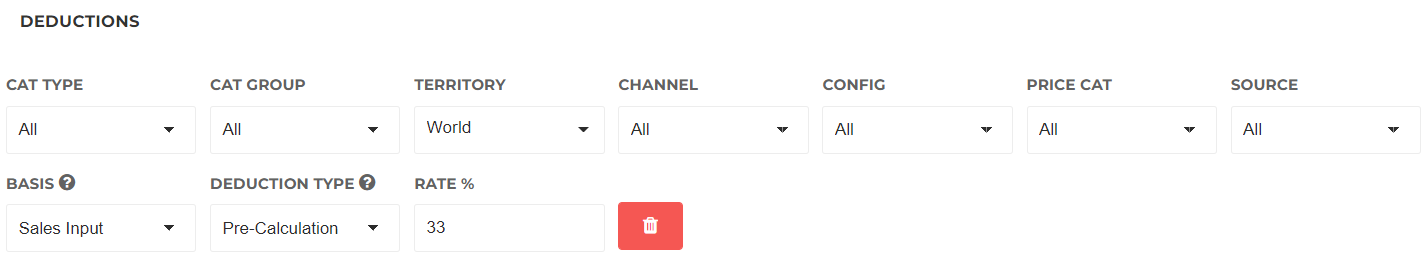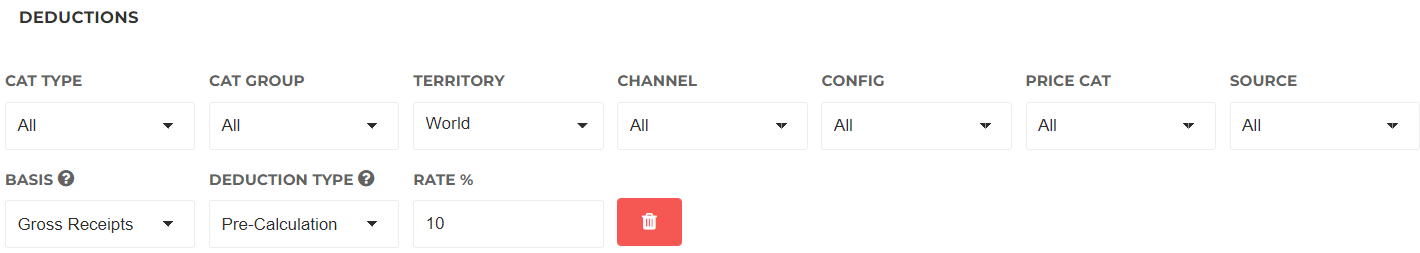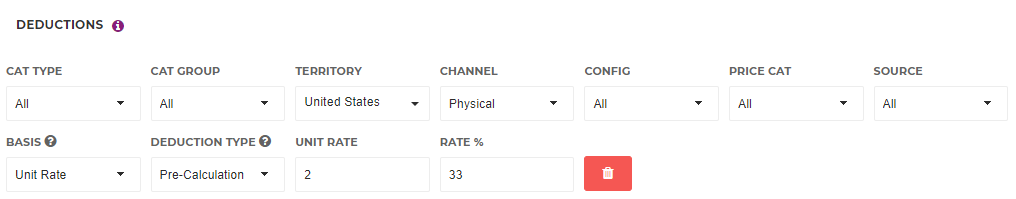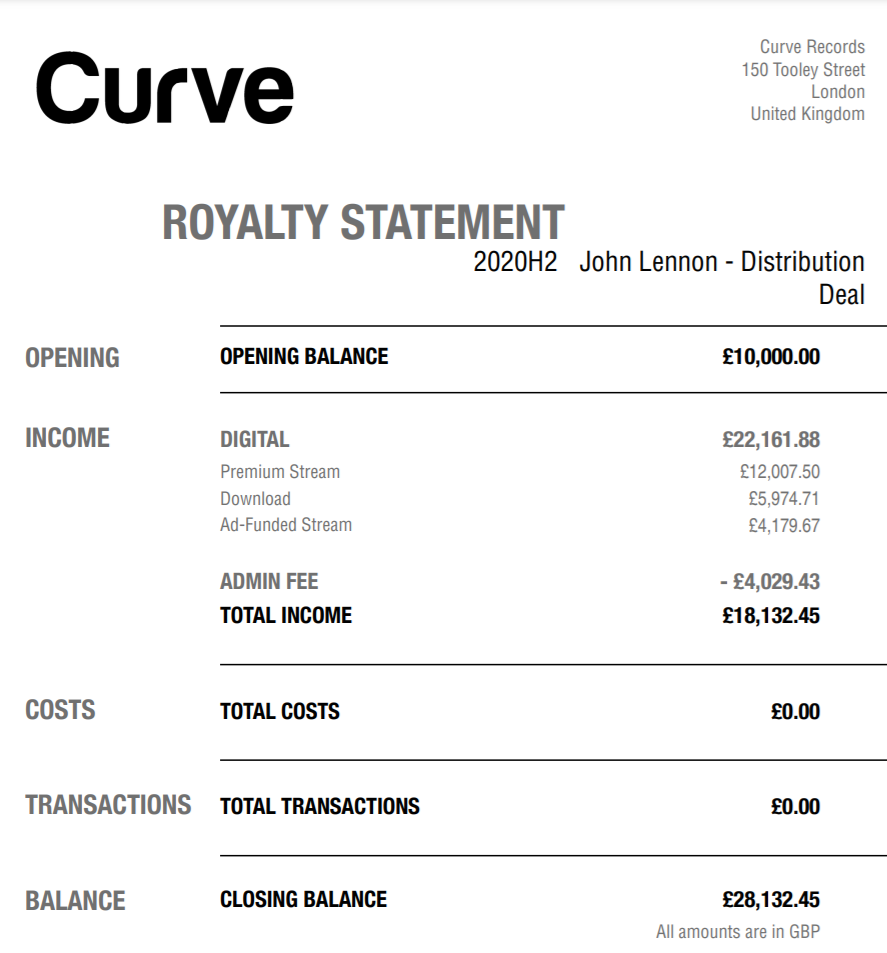Whilst you calculate a royalty for your artist via your Sales Terms, it may be you need to apply a further deduction under certain conditions. Deductions work in a similar fashion to sales terms. You are able to deduct either a percentage of the sales input, or a percentage of a different calculation input basis, or a unit rate. It can be done either before (Pre-), or after (Post-) the sales term calculation that relates to the programmed deduction.

Deductions are available to Curve Pro clients only as part of the Deductions add-on ✪

You can add multiple Deductions to the same Contract. However, only ever one Deduction will be applied. Only the matching Deduction term with the most specific conditions will be applied. The same rules of hierarchy are used for Deduction terms as they do for Sales terms.

Via your Statement Design settings, you can specify to name and display the Deductions in your PDF statements.

## Deduction Basis

### Deductions on the Sales Input

The below example applies a deduction to the royalty calculation. Imagine there is a total of \$100 in Net Receipts, and this Contract has a sales term that applies a 50% royalty to the Net Receipts, then a deduction of 33% will be added to this calculation. In a Pre-Calculation deduction, we will take the calculation input (\$100), apply the Deduction % (\$100 goes down to \$67), and then apply the 50% royalty rate (\$67 goes down to \$33.5).### Deductions with an absolute Calculation Input

Other than applying an additional percentage on the royalty calculation using the Sales Input, you also have the option to apply a deduction based on a percentage of an absolute basis. The basis options are Net Receipts, Gross Receipts, PPD, Unit Price, Retail Price, Unit Rate and Fixed Unit Rate. More info on what these calculation basis can be found here.

In the below example, we apply a deduction equal to 10% of the Gross Receipts. In a scenario where we have \$110 in Gross Receipts, \$100 in Net Receipts, and a royalty rate of 50% of Net Receipts for the artist; we will take the calculation input (\$100), deduct this by the deduction value (10% of \$110 is \$11, so we'll reduce \$100 down to \$89), and then apply the royalty rate (50% of \$89 is \$44.5).The following example is a Unit Rate deduction and deducts 0.66 (33% rate of the unit rate 2) from this artist’s royalty per physical sales unit in the United States. Let’s imagine we sold 10 units in the United States with a total revenue of \$100, with the artist on a royalty rate of 20% of net receipts. In this below example, \$100 will be deducted by \$6.60 (the 10 units multiplied by the deduction unit rate of \$0.66), then calculate the artist’s 20% royalty which results in a bottom line of \$18.68.## Pre-Calculation vs Post-Calculation

In a Pre-Calculation type, we will take the calculation input, reduce this by the deduction value, and then apply the royalty rate. In a Post-Calculation type, we will take the calculation input, apply the royalty rate, and then reduce this by the deduction value.

Please note that in the scenario of a Profit Share deal, all deductions will be applied to the earnings before the Profit Share % is applied.

### In a Sales Input Deduction

We again look at the above three examples, but this time imagine a Post-Calculation deduction type. Let's take a look at our first example.

Pre-Calculation = Calculation Input * Deduction * Royalty Rate = \$100 x (1 - 33%) x 50% = \$33.5
Post-Calculation = Calculation Input * Royalty Rate * Deduction = \$100 x 50% x (1 - 33%) = \$33.5

As you may have noticed, in deductions with a Sales Input basis, the deduction type does not make a difference.

### In an absolute Deduction

It's a different story when we look at deductions with an absolute basis. Let's imagine again our second example, but this time also with a Post-Calculation.

Pre-Calculation = (Calculation Input - Deduction) * Royalty Rate = [\$100 - (\$110 x 10%)] x 50% = \$44.5
Post-Calculation = (Calculation Input * Royalty Rate) - Deduction = [100 x 50%] - [\$110 x 10%] = \$39

You will find that the output of our calculation is now different, because we apply the deduction after the royalty rate is applied. In essence, the deduction value is deducted fully from the artist share.

Let's look at our final example of a Unit Rate deduction, also this time with a Post-Calculation.

Pre-Calculation = (Calculation Input - Deduction) * Royalty Rate = [[\$100 - \$6.60] x 20% = \$18.68
Post-Calculation = (Calculation Input * Royalty Rate) - Deduction = [\$100 x 20%] - \$6.66 = \$13.34

## Displaying the Deductions in your PDF Statements

When you go to your Settings > General > Statement Design options, you will find the option to enable " Display Separated Deductions". This will create a separate line in your PDF statements, highlighting any deductions taken. Via the Deduction Name field, you can specify how these deductions are named in your PDF statement.Below is the example of a Combined statement design for a Royalty style deal. With this option enabled, the Admin Fee deduction is displayed as a separate category in the Income area.